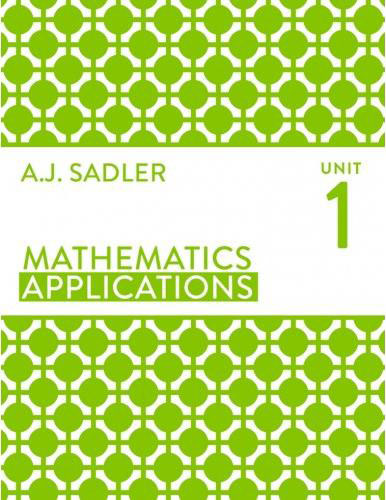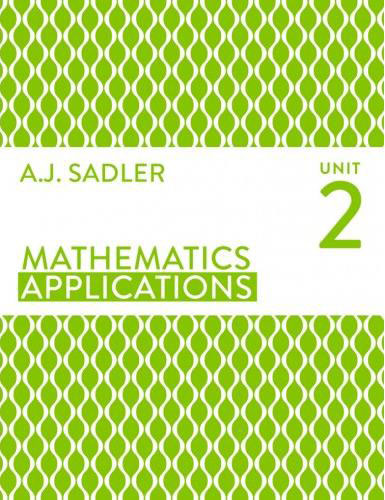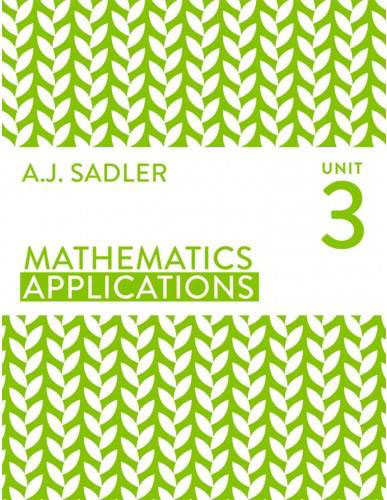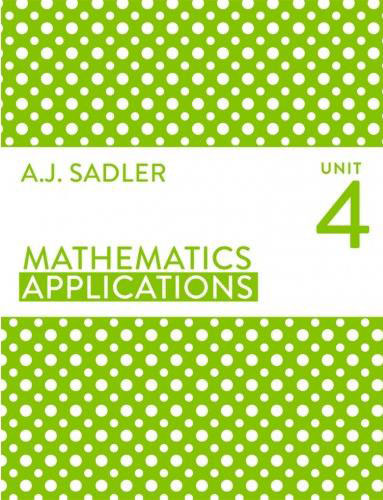## Mathematics Applications

Home / Mathematics Applications

# Mathematics Applications

## Mathematics Applications (ATAR) Tutor Perth

At Tutor Perth we hand pick the finest Maths Applications home tutors in Perth. Our Maths Applications tutors are engaging role models, mentors and are genuine specialists at teaching maths. We teach in accordance to the WA curriculum framework and provide comprehensive tutoring solutions to the A.J Sadler suite of text books, units 1, 2, 3 and 4.

Our engaging maths tutors help students achieve top ATAR results in the following A.J Sadler units:

## Year 11 Mathematics Applications (ATAR) | Specialist Tutors

### We Tutor – Unit 1

• Use of formulae
• Percentages
• Simple interest
• Compound interest
• Other financial considerations
• Matrices
• The theorem of Pythagoras
• Perimeter and area
• Surface area and volume
• Similarity### We Tutor – Unit 2

• Univariate data
• Summarising data and distributions
• Boxplots and histograms
• The statistical investigation process
• Solving equations
• Using equations to solve problems
• Linear relationships
• Piecewise defined relationships
• Trigonometry for right triangles
• Trigonometry for non-right angled triangles
• Simultaneous linear equations
• Standard scores and the normal distribution

## Year 12 Mathematics Applications (ATAR) | Specialist Tutors### We Tutor – Unit 3

• Bivariate data
• Bivariate data – further analysis
• Sequences – recursion
• Networks
• Shortest path

### We Tutor – Unit 4

• Time series data
• Moving averages and seasonal effects
• Finance I: Saving and borrowing
• Minimum spanning trees
• Maximum flow
• Project networks
• Assignment problems